# Namespaces in C++

## Namespaces in C++ with Examples

In this article, I am going to discuss Namespaces in C++ with Examples. Please read our previous article where we discussed Pre-Processor Directives in C++ with Examples.

##### Namespaces in C++:

Namespaces are used for removing name conflicts in C++. If you are writing multiple functions with the same name but are not overloaded, they are independent functions. They are not a part of the class. If you are writing multiple classes that have the same name, then there will be name conflicts.

Let us understand this with Examples. Please have a look at the following code. Here we have a function fun and another function fun, which is of the same name. These are two independent functions. These are not part of the base class or derive class. And these are not overloaded functions. These are global functions. And lastly, we have one main function.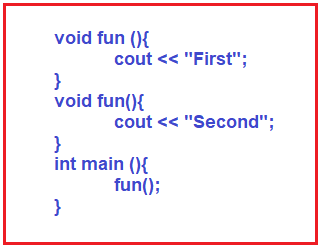So, we have two functions of name fun and one main function. The main function is to call the function fun. So, which fun function will be called? First fun or second fun? First of all, the compiler will not compile our program. The compiler will say that we are redefining the same function multiple times. For a better understanding, please have a look at the following example.

```#include <iostream>
using namespace std;

void fun()
{
cout << "First";
}

void fun()
{
cout << "Second";
}

int main()
{
fun();
return 0;
}
```
###### Output: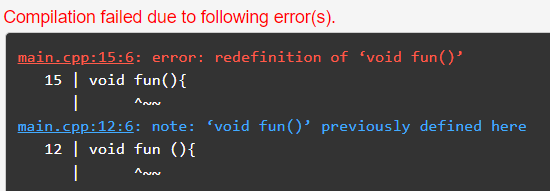But we want the same function but we want to remove this ambiguity. We have to remove the name conflicts. So, for this, we can use namespaces in C++. Let us define our namespace as follows: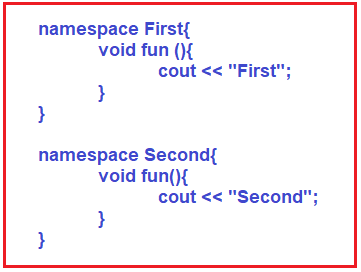So here we have two namespaces that are First and Second in which we have our fun functions. Here we have encapsulated functions inside the namespace. We have written those functions in the namespace. When we call the functions, we must give the namespace before calling the functions. For example,So first we have to write the namespace, then the scope resolution operator, and then the function name. So, the above line will execute two different functions of the same name, fun. So, when you have two functions with the same name, you can separate them. We can introduce a namespace to give them a distinct identity. The complete example code is given below.

```#include <iostream>
using namespace std;

namespace First
{
void fun()
{
cout << "First" <<endl;
}
}
namespace Second
{
void fun()
{
cout << "Second" <<endl;
}
}

int main()
{
First::fun ();
Second::fun ();
return 0;
}
```
###### Output: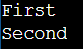##### What are the things we can write inside a namespace in C++?

In one namespace, we can have many things, not just one function. Suppose we have access to all the data and functions related to a class and objects. We can keep them in one namespace. So, we have given an example of having only one function in a namespace. Instead of writing every time namespace and then scope resolution, we can write one statement at the start of the program, that is,

using namespace First;

Now when we call the function fun anywhere in the program, it will call fun inside the first namespace. If you still want to call the second namespace function, then you can write,

Second::fun();

For a better understanding, please have a look at the following example which shows the above-discussed two points.

```#include <iostream>
using namespace std;

namespace First
{
void fun()
{
cout << "First" <<endl;
}
}
namespace Second
{
void fun()
{
cout << "Second" <<endl;
}
}

using namespace First;
int main()
{
fun();
Second::fun();
return 0;
}
```
###### Output: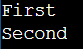In our C++ program, we have been using namespace std, so there is one namespace std that has the cin and cout objects. So that’s why we just write a single line using namespace std; and we can use cin and cout objects. Otherwise, we have to write like this,

std::cout << “Hello”;

The complete example is given below.

```#include <iostream>
int main()
{
std::cout << "Hello";
return 0;
}
```

Output: Hello

To avoid writing the namespace name, again and again, we just write a single statement at the start of the C++ program. We can keep these namespaces in separate header files, and then we can include that header file in our C++ program.